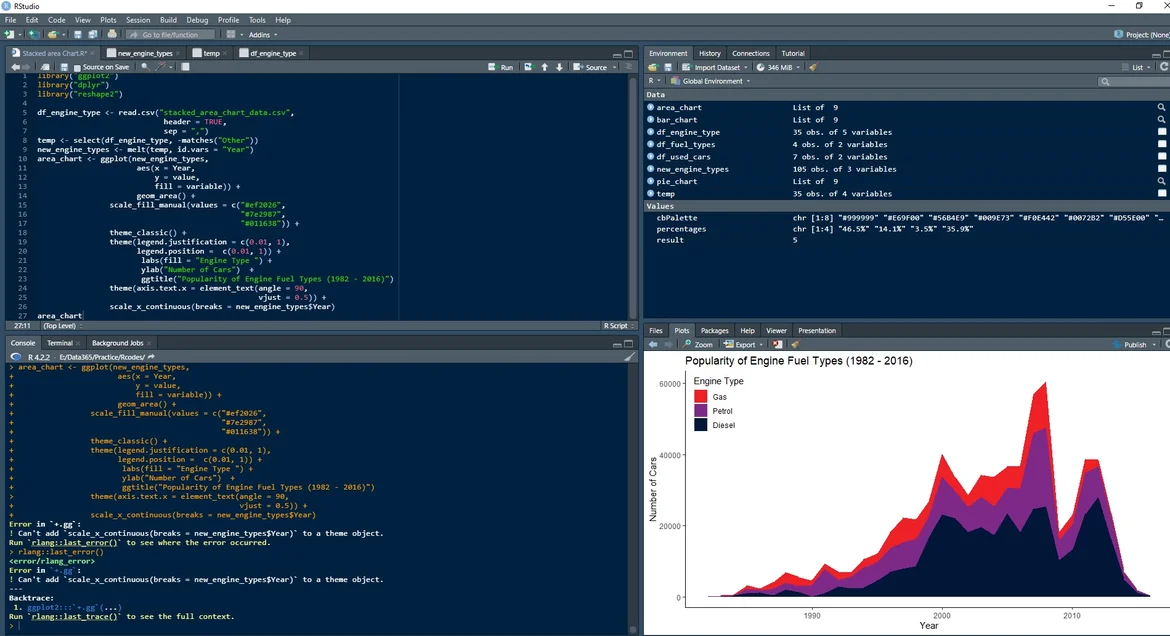Super learner

30 Jan 2023

Posted on:

12 Dec 2022

0

# Resolved:Having an error in line 26

I am getting an error in Line 26 and cant figure out what is wrong with it

``````library("ggplot2")
library("dplyr")
library("reshape2")

sep = ",")
temp <- select(df_engine_type, -matches("Other"))
new_engine_types <- melt(temp, id.vars = "Year")
area_chart <- ggplot(new_engine_types,
aes(x = Year,
y = value,
fill = variable)) +
geom_area() +
scale_fill_manual(values = c("#ef2026",
"#7e2987",
"#011638")) +
theme_classic() +
theme(legend.justification = c(0.01, 1),
legend.position =  c(0.01, 1)) +
labs(fill = "Engine Type ") +
ylab("Number of Cars")  +
ggtitle("Popularity of Engine Fuel Types (1982 - 2016)")
theme(axis.text.x = element_text(angle = 90,
vjust = 0.5)) +
scale_x_continuous(breaks = new_engine_types\$Year)
area_chart
``````

Console shows

``````+                 scale_x_continuous(breaks = new_engine_types\$Year)
Error in `+.gg`:
! Can't add `scale_x_continuous(breaks = new_engine_types\$Year)` to a theme object.
Run `rlang::last_error()` to see where the error occurred.
``````

Super learner
Posted on:

12 Dec 2022

0Super learner
Posted on:

12 Dec 2022

0

Figured it out.
How do I delete thread If I found out answer before any replay?

Instructor
Posted on:

30 Jan 2023

0

Hi Valdas,